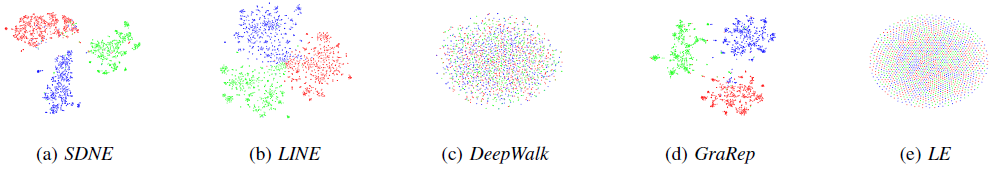0%

# Structural Deep Network Embedding

## 简介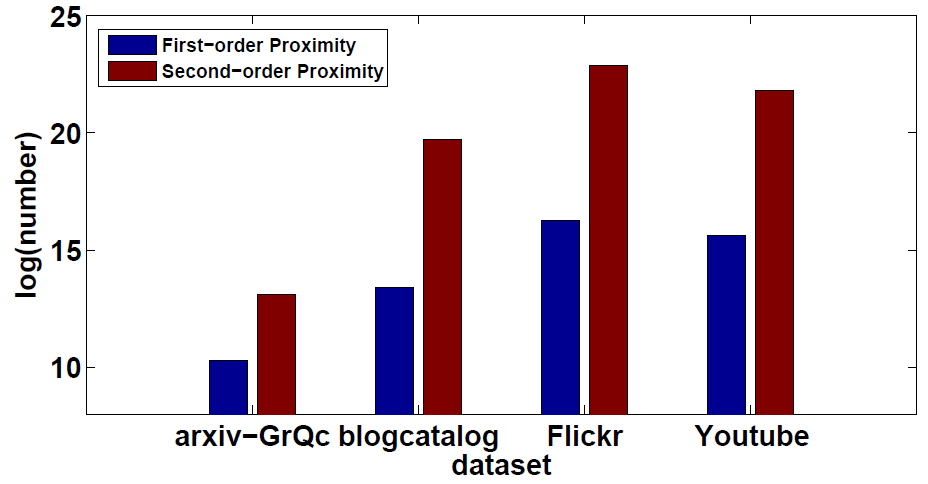### 主要贡献

1. 我们提出了一种深层网络结构嵌入的方法，称为SDNE。这种方法能够将网络数据映射到深层的非线性低维空间，并且具有较好的鲁棒性。同时具我们所知，该方法是第一次将深度学习运用于网络表示中。
2. 我们提出了一个新的半监督深层模型，整合了网络中的一阶和二阶相似性。因此，通过该模型得到的低维网络表示，能够很好的表现网络的局部和整体特征。
3. 我们提出的算法在5个真实的数据集中，分别对2种应用问题（多标签分类、可视化）进行了实验验证。结果显示，对于网络标签稀少的数据，我们比其它基准方法提升了至少20%的效果。在某些情况下，我们只需要60%甚至更少的训练数据，也能得到很好的成绩。

### 其他相关工作

#### IsoMAP

1. 通过k-Nearest neighbor算法得到每个点的一个近邻。（参数k）
2. 通过最短路算法构造一个N*N的距离矩阵。
3. 通过Multi-dimensional Scaling算法根据距离矩阵进行非线性降维。（参数e）
算法结束以后，我们得到的就是一些e维空间的点。

#### DeepWalk

Gram训练每一个节点的向量。

$$f = \frac{1}{{\left| S \right|}}\sum\limits_{i = 1}^{\left| S \right|} {\sum\limits_{ - w \le j \le w,j \ne 0} {\log p({v_{i + j}}|{v_i})} }$$

$$p\left( {{v_j}|{v_i}} \right) = \frac{{exp\left( {c_{{v_j}}^T{r_{{v_i}}}} \right)}}{{\sum\nolimits_{v \in C} {exp\left( {c_{{v_j}}^T{r_{{v_i}}}} \right)} }}$$ ${r_{{v_i}}}$是点${v_i}$的向量表征, ${c_{{v_i}}}$是点${v_i}$上下文中点${v_j}$的向量表征。

DeepWalk使目标$f$最大化，使用Skip-Gram与Hierarchical Softmax进行训练得到每个点的vector，DeepWalk等价于MF(matrix factorization,矩阵分解)。

## 深层网络结构嵌入

### SNDE模型

#### 算法框架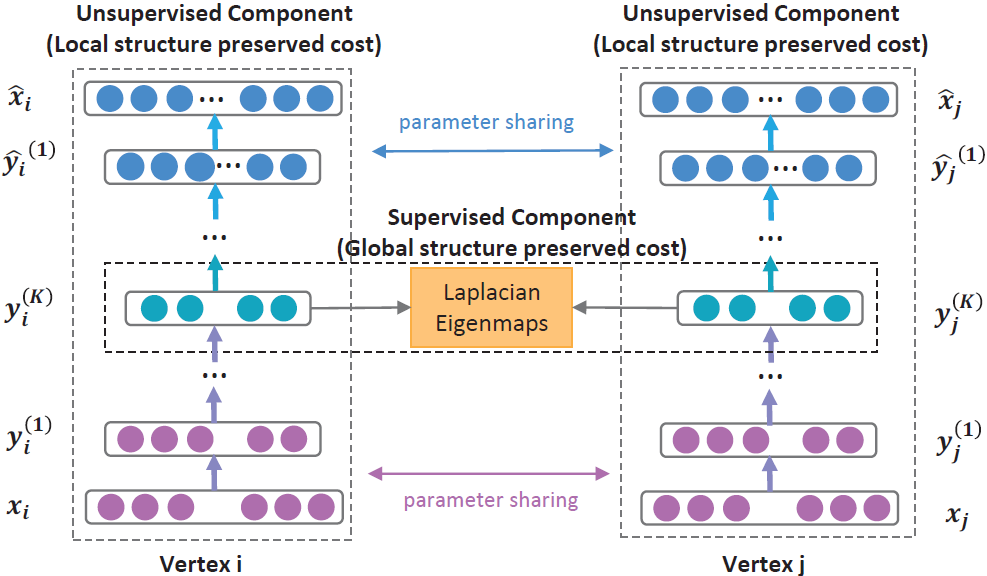### 损失函数

$$y_i^{(1)} = \sigma ({W^{(1)}}{x_i} + {b^{(1)}})$$ $$y_i^{(k)} = \sigma ({W^{(k)}}y_i^{(k - 1)} + {b^{(k)}}),k = 2, \cdots ,K$$

$${\rm{\mathcal{L}}} = \sum\limits_{i = 1}^n {\left\| {{{\hat x}_i} - {x_i}} \right\|_2^2}$$

$$\begin{array}{l}{{\rm{\mathcal{L}}}_{2nd}} = \sum\limits_{i = 1}^n {\left\| {({{\hat x}_i} - {x_i}) \odot {b_i}} \right\|_2^2} \\{\kern 1pt} {\kern 1pt} {\kern 1pt} {\kern 1pt} {\kern 1pt} {\kern 1pt} {\kern 1pt} {\kern 1pt} {\kern 1pt} {\kern 1pt} {\kern 1pt} {\kern 1pt} {\kern 1pt} {\kern 1pt} {\kern 1pt} {\kern 1pt} {\kern 1pt} {\kern 1pt} {\kern 1pt} {\kern 1pt} {\kern 1pt} {\kern 1pt} = \left\| {(\hat X - X) \odot B} \right\|_F^2\end{array}$$

$$\begin{array}{l}{{\rm{\mathcal{L}}}_{1nd}} = \sum\limits_{i = 1}^n {{s_{i,j}}\left\| {y_i^{(K)} - y_j^{(K)}} \right\|_2^2} \\{\kern 1pt} {\kern 1pt} {\kern 1pt} {\kern 1pt} {\kern 1pt} {\kern 1pt} {\kern 1pt} {\kern 1pt} {\kern 1pt} {\kern 1pt} {\kern 1pt} {\kern 1pt} {\kern 1pt} {\kern 1pt} {\kern 1pt} {\kern 1pt} {\kern 1pt} {\kern 1pt} {\kern 1pt} {\kern 1pt} {\kern 1pt} {\kern 1pt} = \sum\limits_{i = 1}^n {{s_{i,j}}\left\| {{y_i} - {y_j}} \right\|_2^2} \end{array}$$

$$\begin{array}{l}{{\rm{\mathcal{L}}}_{mix}} = {{\rm{\mathcal{L}}}_{2nd}} + \alpha {{\rm{\mathcal{L}}}_{1nd}} + \upsilon {{\rm{\mathcal{L}}}_{reg}}\\{\kern 1pt} {\kern 1pt} {\kern 1pt} {\kern 1pt} {\kern 1pt} {\kern 1pt} {\kern 1pt} {\kern 1pt} {\kern 1pt} {\kern 1pt} {\kern 1pt} {\kern 1pt} {\kern 1pt} {\kern 1pt} {\kern 1pt} {\kern 1pt} {\kern 1pt} {\kern 1pt} {\kern 1pt} {\kern 1pt} {\kern 1pt} {\kern 1pt} = \left\| {(\hat X - X) \odot B} \right\|_F^2 + \alpha \sum\limits_{i = 1}^n {{s_{i,j}}\left\| {{y_i} - {y_j}} \right\|_2^2} + \upsilon {{\rm{\mathcal{L}}}_{reg}}\end{array}$$

$$\begin{array}{l}{{\rm{\mathcal{L}}}_{mix}} = {{\rm{\mathcal{L}}}_{2nd}} + \alpha {{\rm{\mathcal{L}}}_{1nd}} + \upsilon {{\rm{\mathcal{L}}}_{reg}}\\{\kern 1pt} {\kern 1pt} {\kern 1pt} {\kern 1pt} {\kern 1pt} {\kern 1pt} {\kern 1pt} {\kern 1pt} {\kern 1pt} {\kern 1pt} {\kern 1pt} {\kern 1pt} {\kern 1pt} {\kern 1pt} {\kern 1pt} {\kern 1pt} {\kern 1pt} {\kern 1pt} {\kern 1pt} {\kern 1pt} {\kern 1pt} {\kern 1pt} = \left\| {(\hat X - X) \odot B} \right\|_F^2 + \alpha \sum\limits_{i = 1}^n {{s_{i,j}}\left\| {{y_i} - {y_j}} \right\|_2^2} + \upsilon {{\rm{\mathcal{L}}}_{reg}}\end{array}$$

## 实验

### 数据集

BLOGCATALOG 10312 667966
FLICKR 80513 11799764
ARXIV GR-QC 5242 28980
20-NEWSGROUP 1720 Full-connected

### 评价指标

$$Macro - F1 = \frac{{\sum\nolimits_{A \in {\rm{\mathcal{C}}}} {F1(A)} }}{{\left| {\rm{\mathcal{C}}} \right|}}$$

Micro-F1是对每个实例权重的度量。定义如下：

$$\Pr = \frac{{\sum\nolimits_{A \in {\rm{\mathcal{C}}}} {TP(A)} }}{{\sum\nolimits_{A \in {\rm{\mathcal{C}}}} {(TP(A) + FP(A))} }}$$ $$R = \frac{{\sum\nolimits_{A \in {\rm{\mathcal{C}}}} {TP(A)} }}{{\sum\nolimits_{A \in {\rm{\mathcal{C}}}} {(TP(A) + FN(A))} }}$$ $$Micro - F1 = \frac{{2*\Pr *R}}{{\Pr + R}}$$

### 参数设置

BLOGCATALOG 10300-1000-100
FLICKR 80513-5000-1000-100
ARXIV GR-QC 5242-500-100
20-NEWSGROUP 1720-200-100

### 实验结果

#### 多标签分类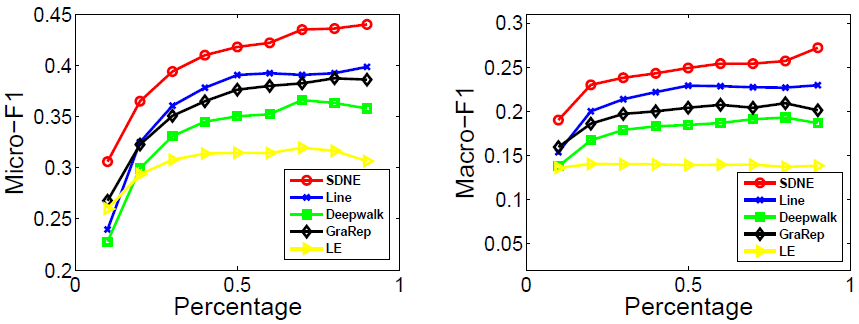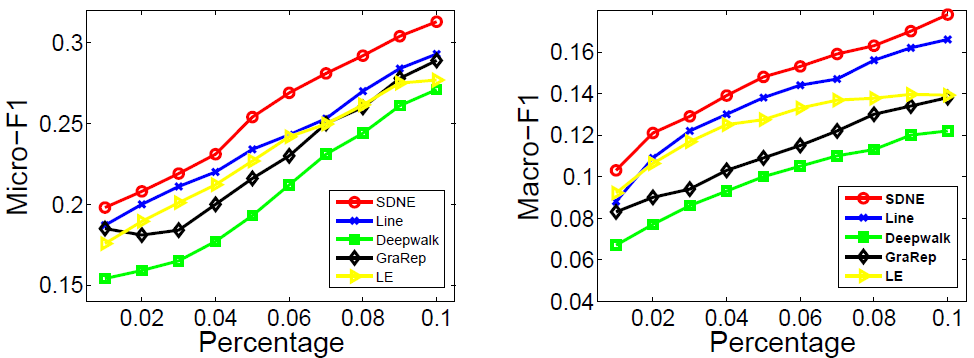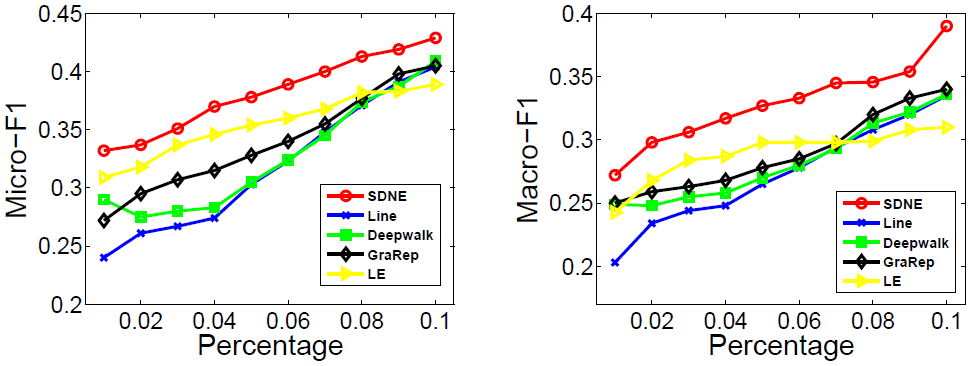#### 可视化